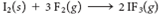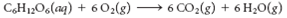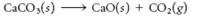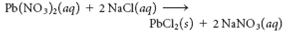Problem

# For each of the following processes, specify whether entropy increases or decreases. Expla...

For each of the following processes, specify whether entropy increases or decreases. Explain each of your answers.

(a) Assembling a jigsaw puzzle

(b)(c) A precipitate forming when two solutions are mixed

(d)(e)(f)#### Step-by-Step Solution

Solution 1

a) When a jigsaw puzzle assembled, disorderness decreases.

So entropy decreases.

b) Given equation: I₂(s)+3 F₂(g) ⟶ 2 IF₃(g)
Entropy deceases because products ( 2 moles of gas) are less disordered than reactants ( 1 mol of solid +3 moles of gas).

c) When a precipitate formed from mixing of two solutions, disorderness decreases.

So entropy decreases.

d) Given equation: C₆H12O₆(a q)+6O₂(g) ⟶ 6CO₂(g)+6 H₂O(g)
Entropy increases because products (12 moles of gas) are more disordered than reactants (1) mole of liquid +6 moles of gas)
e) Given equation: CaCO₃(s) ⟶ CaO(s)+CO₂(g)
Entropy increases because products (1 mole of soild +1 mole of gas) are more disordered than reactants (1 molot solid)
f) Given equation: Pb(NO₃)₂(a q)+2 NaCl(a q) ⟶ PbCl₂(s)+2 NaNO₃(a q)
Entropy decreases because products ( 1 mol of solid +2 moles of liquid) are less disordered than reactants ( 3 moles of liquid)